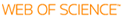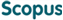### BROWSE

An efficient numerical approach for solving caputo fractional nonlinear two-point boundary value problems

Cited 0 times inCited 0 times inTitle
An efficient numerical approach for solving caputo fractional nonlinear two-point boundary value problems
Author
Keywords
Capuoto fractional derivative; Fractional differential equation; Fractional order boundary value problem; predictor-corrector methods; Nonlinear shooting methods
Issue Date
2020-08
Publisher
Abstract
This paper proposed a new type of numerical scheme for solving Multi-term Fractional Nonlinear Differential Equation with Two Point Boundary Value Problems(FBVP). The proposed methods take lower computational cost than conventional methods. In new techniques, the FBVP transposes to the System of Fractional Nonlinear Initial Value Problems(FIVP). In order to solve the system of FIVP, the Higher-Order Predictor-Corrector Method(HOM) is applied. Moreover, we employ shooting method based on Newton's and Halley's methods to approximate the unknown initial values of the system. Several numerical experiments show that the proposed methods give the same rate of convergence in the HOM.
Description
Department of Mathematical Sciences
URI